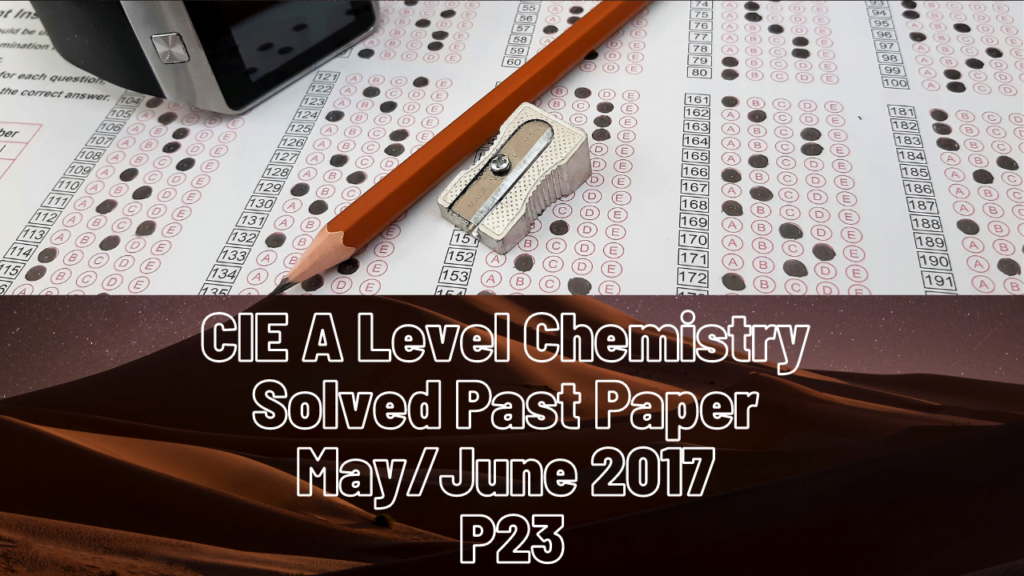A Level Chemistry### CIE A Level Chemistry Solved Past Paper May/June 2017 P23

1a) Isomer with the same molecular formula and different structural formula (position, functional group, or branch)

b(i)   Volume of CO2 formed = 150-50=100cm3, from 25cm3 reactant

Simplify to get x=4

(ii) Volume of reacting O2 = 200-50 =250cm3

(iii) C4H8   CH2

(iv) But-1-ene and but-2-ene

(v) Mass = (100000*56*25/10^6)/ (8.31*37+273) = 0.0543

2a(i)   Green gas, brown liquid, grey solid.

(ii) Volatility is dependent on intermolecular forces. Going down the group, the number of electrons increases. This increases the strength of intermolecular forces due to temporary dipole-induced dipoles, hence reducing volatility.

b) It decreases. Going down the group, the number of shells increases. This increases the shielding effect and decreases nuclear attraction for electrons.

C(i) White fumes, purple vapour

(ii) Sulfuric acid acts as an oxidizing agent with iodine because iodine is a weaker oxidizing agent. Chlorine is a stronger oxidizing agent than sulfuric acid and so t=does not get oxidized.

(iii) Sulfuric acid+ sodium bromide —- sodium hydrogen sulphate + hydrogen bromide

hydrogen bromide + sulfuric acid = bromine + water + sulfur dioxide

d(i) Silver iodide

(ii) Silver chloride dissolves in ammonia, and silver iodide remains insoluble.

3a(i) The enthalpy change that occurs when 1 mole of a compound is formed from its elements in their standard states under standard conditions.

a(ii) x = -394.8

b(i) Label further ahead of the label in question.

(ii) Rate of reaction increases when catalyst provides alternates route. More of the reactant particles have energy greater than activation energy. This leads to higher frequency of successful collisions

(iii) Rate increases. Reactant particles are closer together, meaning collisions per unit volume increase. Yield increases. The product side of the equation has fewer molecules. Equilibrium d=shifts to right hand side.

C(i) 0.01, 0.99

(ii) 50300

d(i) Divide products by reactants’ partial pressures.